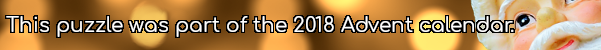mscroggs.co.ukmscroggs.co.uksubscribe

## 8 DecemberArrange the digits 1-9 in a 3×3 square so: each digit the first row is the number of letters in the (English) name of the previous digit, each digit in the second row is one less than the previous digit, each digit in the third row is a multiple of the previous digit; the second column is an 3-digit even number, and the third column contains one even digit. The number in the first column is today's number.
 each digit is the number of letters in the previous digit each digit is one less than previous each digit is multiple of previous today's number even 1 even digit
Edit: There was a mistake in this puzzle: the original had two solutions. If you entered the wrong solution, it will automatically change to the correct one.
Tags: numbers, grids

## Archive

Show me a random puzzle
▼ show ▼